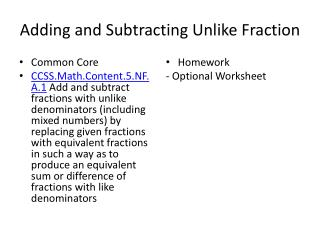DownloadDownload Presentation# Adding and Subtracting Unlike Fraction

Download Presentation## Adding and Subtracting Unlike Fraction

- - - - - - - - - - - - - - - - - - - - - - - - - - - E N D - - - - - - - - - - - - - - - - - - - - - - - - - - -
##### Presentation Transcript

1. Adding and Subtracting Unlike Fraction • Common Core • CCSS.Math.Content.5.NF.A.1 Add and subtract fractions with unlike denominators (including mixed numbers) by replacing given fractions with equivalent fractions in such a way as to produce an equivalent sum or difference of fractions with like denominators • Homework - Optional Worksheet

2. Adding and Subtracting Fractions • Draw picture models to solve the problems -Alex squeezed 2 liters of juice for breakfast. If he pours the juice equally into 5 glasses, how many liters of juice will be in each glass?

3. Picture models • Draw a picture model to show -